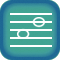Intervals II: Seconds and Thirds

Skills covered:
 Major and Minor SecondsMajor and Minor Thirds The Tritone IntervalPerfect Intervals

1.  Major and minor seconds
Lesson

2.  Aural recognition of m2, M2, P4 and P8 (melodic)
Lesson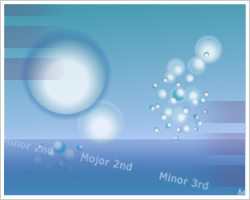Melodic Drops
Level 4

3.  Aural recognition of m2, M2, P4 and P8 (harmonic)
Lesson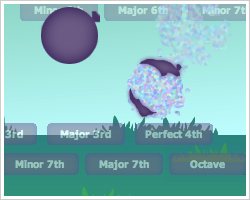Harmonic Drops
Level 4

4.  M2, P4, P5 and P8 in major keys (solfege)
LessonTwo Tones (Major)
Level 4

5.  M2, P4, P5 and P8 in minor keys (solfege)
LessonTwo Tones (Minor)
Level 1

6.  m2, P4, P5 and P8 in major keys (solfege)
LessonTwo Tones (Major)
Level 5

7.  m2, P4, P5 and P8 in minor keys (solfege)
LessonTwo Tones (Minor)
Level 2

8.  Notation of M2, P4, P5 and P8
Lesson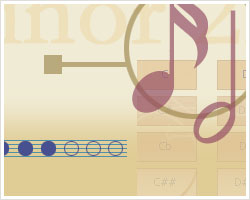Flash Notation (Intervals)
Level 2

9.  Notation of m2, P4, P5 and P8
LessonFlash Notation (Intervals)
Level 3

10.  Aural recognition of M2, P4, P5 and P8 (melodic)
Lesson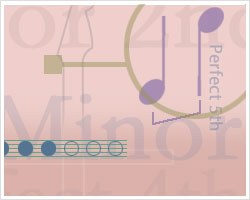Flash Intervals (Mel.)
Level 2

11.  Aural recognition of M2, P4, P5 and P8 (harmonic)
Lesson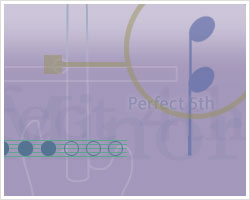Flash Intervals (Harmonic)
Level 2

12.  Aural recognition of m2, P4, P5 and P8 (melodic)
LessonFlash Intervals (Mel.)
Level 3

13.  Aural recognition of m2, P4, P5 and P8 (harmonic)
LessonFlash Intervals (Harmonic)
Level 3

14.  Aural recognition of m2, M2, P4, P5 and P8 (melodic) 1
LessonMelodic Drops
Level 5

15.  Aural recognition of m2, M2, P4, P5 and P8 (harmonic) 1
LessonHarmonic Drops
Level 5

16.  m2, M2, P4, P5 and P8 in major keys (solfege)
LessonTwo Tones (Major)
Level 6

17.  m2, M2, P4, P5 and P8 in minor keys (solfege)
LessonTwo Tones (Minor)
Level 3

18.  Notation of m2, M2, P4, P5 and P8
LessonFlash Notation (Intervals)
Level 4

19.  Aural recognition of m2, M2, P4, P5 and P8 (melodic) 2
LessonFlash Intervals (Mel.)
Level 4

20.  Aural recognition of m2, M2, P4, P5 and P8 (harmonic) 2
LessonFlash Intervals (Harmonic)
Level 4

21.  Major and minor thirds
Lesson

22.  Aural recognition of m3, M3, P5 and P8 (melodic)
LessonMelodic Drops
Level 6

23.  Aural recognition of m3, M3, P5 and P8 (harmonic)
LessonHarmonic Drops
Level 6

24.  M3, P4, P5 and P8 in major keys (solfege)
LessonTwo Tones (Major)
Level 7

25.  m3, P4, P5 and P8 in minor keys (solfege)
LessonTwo Tones (Minor)
Level 4

26.  Notation of M3, P4, P5 and P8
LessonFlash Notation (Intervals)
Level 5

27.  Notation of m3, P4, P5 and P8
LessonFlash Notation (Intervals)
Level 6

28.  Aural recognition of M3, P4, P5 and P8 (melodic)
LessonFlash Intervals (Mel.)
Level 5

29.  Aural recognition of M3, P4, P5 and P8 (harmonic)
LessonFlash Intervals (Harmonic)
Level 5

30.  Aural recognition of m3, P4, P5 and P8 (melodic)
LessonFlash Intervals (Mel.)
Level 6

31.  Aural recognition of m3, P4, P5 and P8 (harmonic)
LessonFlash Intervals (Harmonic)
Level 6

32.  Aural recognition of m3, M3, P4, P5, and P8 (melodic) 1
LessonMelodic Drops
Level 7

33.  Aural recognition of m3, M3, P4, P5, and P8 (harmonic) 1
LessonHarmonic Drops
Level 7

34.  Notation of m3, M3, P4, P5 and P8
LessonFlash Notation (Intervals)
Level 7

35.  Aural recognition of m3, M3, P4, P5, and P8 (melodic) 2
LessonFlash Intervals (Mel.)
Level 7

36.  Aural recognition of m3, M3, P4, P5, and P8 (harmonic) 2
LessonFlash Intervals (Harmonic)
Level 7

37.  Aural recognition of m2, M2, m3 and M3 (melodic) 1
LessonMelodic Drops
Level 8

38.  Aural recognition of m2, M2, m3 and M3 (harmonic) 1
LessonHarmonic Drops
Level 8

39.  m2, M2, m3 and M3 in major keys (solfege)
LessonTwo Tones (Major)
Level 8

40.  m2, M2, m3, M3 in minor keys (solfege)
LessonTwo Tones (Minor)
Level 5

41.  Notation of m2, M2, m3 and M3
LessonFlash Notation (Intervals)
Level 8

42.  Aural recognition of m2, M2, m3, M3 (melodic) 2
LessonFlash Intervals (Mel.)
Level 8

43.  Aural recognition of m2, M2, m3 and M3 (harmonic) 2
LessonFlash Intervals (Harmonic)
Level 8

44.  The diminished fifth (tritone)
Lesson

45.  Aural recognition of m2, M2, m3, M3 and d5 (melodic) 1
LessonMelodic Drops
Level 9

46.  Aural recognition of m2, M2, m3, M3 and d5 (harmonic) 1
LessonHarmonic Drops
Level 9

47.  m2, M2, m3, M3 and tritone in major keys (solfege)
LessonTwo Tones (Major)
Level 9

48.  m2, M2, m3, M3 and tritone in minor keys (solfege)
LessonTwo Tones (Minor)
Level 6

49.  m2, M2, m3, M3, P4 and d5 in minor keys (solfege)
LessonTwo Tones (Minor)
Level 7

50.  m2, M2, m3, M3, P4, d5 and P5 in minor keys (solfege)
LessonTwo Tones (Minor)
Level 8

51.  Notation of m2, M2, m3, M3 and tritone
LessonFlash Notation (Intervals)
Level 9

52.  Aural recognition of m2, M2, m3, M3 and d5 (melodic) 2
LessonFlash Intervals (Mel.)
Level 9

53.  Aural recognition of m2, M2, m3, M3 and d5 (harmonic) 2
LessonFlash Intervals (Harmonic)
Level 9

54.  Aural recognition of m2, M2, m3, M3, P4, d5, P5 and P8 (melodic) 1
LessonMelodic Drops
Level 10

55.  Aural recognition of m2, M2, m3, M3, P4, d5, P5 and P8 (harmonic) 1
LessonHarmonic Drops
Level 10

56.  m2, M2, m3, M3, P4, d5, P5, and P8 in major keys (solfege)
LessonTwo Tones (Major)
Level 10

57.  m2, M2, m3, M3, P4, d5, P5 and P8 in minor keys (solfege) 1
LessonTwo Tones (Minor)
Level 9

58.  m2, M2, m3, M3, P4, d5, P5 and P8 in minor keys (solfege) 2
LessonTwo Tones (Minor)
Level 10

59.  Notation of m2, M2, m3, M3, P4, d5, P5 and P8
LessonFlash Notation (Intervals)
Level 10

60.  Aural recognition of m2, M2, m3, M3, P4, d5, P5 and P8 (melodic) 2
LessonFlash Intervals (Mel.)
Level 10

61.  Aural recognition of m2, M2, m3, M3, P4, d5, P5 and P8 (harmonic) 2
LessonFlash Intervals (Harmonic)
Level 10